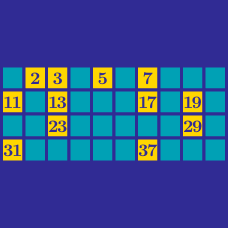Number Theory

# Prime Numbers: Level 3 Challenges

$2^{2014}+ 1007^4$ Is this sum equal to a prime number?

Mr. White is an approximately forty years old father with 4 sons of distinct ages. Writing his age 3 times in succession, we get a 6-digit number that is equal to the product of his age, his wife's age and his 4 sons' ages.

Give the sum of his wife's age and all 4 sons' ages.

A three-digit number $N$ has first digit $a (\neq 0)$, second digit $b$ and third digit $c$. $N = b(10c + b)$ where $b$ and $(10c + b)$ are primes. Find $N$.

How many integers from $1$ to $100$ inclusive can be written as the product of two (not necessarily distinct) primes?

If you can find a computer science or combinatorics approach, post your solution!

What is the last digit of the largest prime number?

See Part 1, and Part 3.

×

Problem Loading...

Note Loading...

Set Loading...